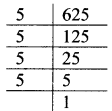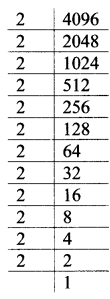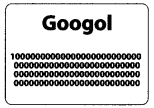# Maharashtra Board Practice Set 30 Class 7 Maths Solutions Chapter 6 Indices

Balbharti Maharashtra State Board Class 7 Maths Solutions covers the 7th Std Maths Practice Set 30 Answers Solutions Chapter 6 Indices.

## Indices Class 7 Practice Set 30 Answers Solutions Chapter 6

Question 1.
Find the square root:
i. 625
ii. 1225
iii. 289
iv. 4096
v. 1089
Solution:
i. 625∴ 625 = 5 x 5 x 5 x 5
∴ √625 = 5 x 5 = 25

ii. 1225∴ 1225 = 5 x 5 x 7 x 7
∴ √1225 = 5 x 7 = 35

iii. 289∴ 289 = 17 x 17
∴ √289 = 17

iv. 4096∴ 4096 = 2 x 2 x 2 x 2 x 2 x 2 x 2 x 2 x 2 x 2 x 2 x 2
∴ √4096 = 2 x 2 x 2 x 2 x 2 x 2
= 64

v. 1089∴ 1089 = 3 x 3 x 11 x 11
∴ √1089 = 3 x 11
= 33

Maharashtra Board Class 7 Maths Chapter 6 Indices Practice Set 30 Intext Questions and Activities

Question 1.
Try to write the following numbers in the standard form. (Textbook pg. no. 48)
i. The diameter of Sun is 1400000000 m.
ii. The velocity of light is 300000000 m/sec.
Solution:
i. 1400000000 m = 1.4 x 109 m
ii. 300000000 m/s = 3.0 x 108 m/sec.

Question 2.
The box alongside shows the number called Googol. Try to write it as a power of 10. (Textbook pg. no. 48)Solution:
1 x 10100

Scroll to Top